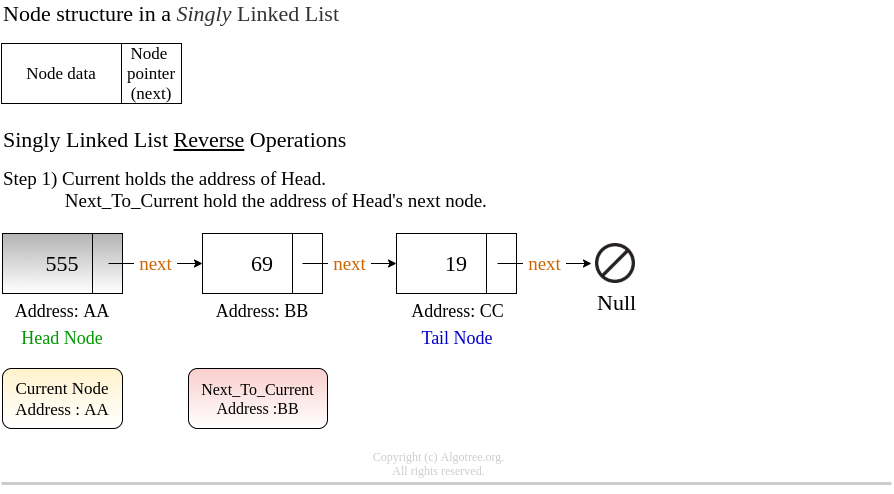# Reversing a singly linked list

Idea to reverse a singly linked list is a below.

a) If there is just a one node in a singly linked list, we return it as it is as there aren’t any links to be reversed.
b) If there are more than one nodes in a singly linked list, we do the following..• Point Head’s next to Null.
• Store the address of the node next to next_to_current into a temp node before reversing the link of next_to_current.
• Point next_to_current to current thus reversing the links between them.
• current takes the place of next_to_current & next_to_current takes place of temp as the reversal of the links happen between every pair of adjacent nodes.

Time Complexity : O ( N ), where N is the number of nodes in the linked list.
Space Complexity : O ( N ), where N is the number of nodes in the linked list.

C++ program to reverse a singly linked list.

``````#include<iostream>

using namespace std;

class Node {

public:
int data;
Node * next;
Node (int arg_data) : data (arg_data), next (nullptr)
{}
};

public:

{}

void Display (Node * head) {

while (current != nullptr) {
cout << current->data << " ";
current = current->next;
}
}

Node * Reverse (Node * head) {

while (next_to_current != nullptr) {

Node * temp = next_to_current->next;
next_to_current->next = current;

current = next_to_current;
next_to_current = temp;
}
return current;
}

}
}
};

int main() {

Node * head = new Node(2);
Node * node_3 = new Node(3);
Node * node_5 = new Node(5);
Node * node_7 = new Node(7);
Node * node_11 = new Node(11);
Node * node_13 = new Node(13);

node_3->next = node_5;
node_5->next = node_7;
node_7->next = node_11;
node_11->next = node_13;

cout <<"\nSingle linked list : " << endl;
cout <<"\nReversing the single linked list : " << endl;
cout <<"\nFreeing the single linked list." << endl;

return 0;
}
``````

Output

``````Single linked list :
2 3 5 7 11 13
Reversing the single linked list :
13 11 7 5 3 2